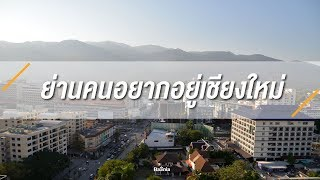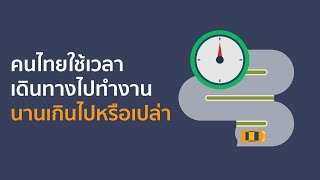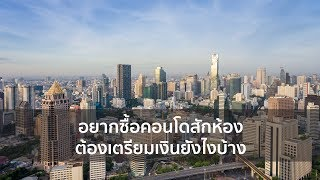•••••••••••••••••••••• เปิดขายกรุงเทพมหานคร · บางซื่อ · บางซื่อ

# Sale Downpayment Regent Home Bangson 28 Available Foreigner Quota (Owner...

ราคา
฿999,900
บันทึก
Share

## รายละเอียด

##### Sale Downpayment Regent Home Bangson 28 Available Foreigner Quota (Owner Post)

Sale Downpayment Regent Home Bangson 28 Available Foreigner Quota (Owner Post)

🔰 Available Thai / Foreigner Quota

⚠️ Can see the real room and ask to see the contract

💵 Deposit 10,000 baht / room (Before deposit, I will send the contract to see first)

✅ The project is located in Soi Bangkok-Nonthaburi 23/1

✅ Close to the Purple Line Sky Train, Bang Son Station, only 100 meters away

✅Shuttle Service to in front of the project

✅ Close to the Si Rat - Outer Ring Road Expressway, just 1.9 km.

🔘 Room size 28 sqm. 56 sq.m. and 61 sq.m.

🔘 Fitness, swimming pool (common area, 30 baht/sq.m.)

🔘 White plaster room walls, sliding glass bathroom partition , Laminate wood, glass, kitchen partition, balcony, exhaust fan

🔘 Parking 1 car per room and 2 cars for Combined rooms

🔘 Key card entry-exit and floor-locked elevators

🔘 Security guards and CCTV 24 hours

••• Building A •••

0405 = 1,140,000

0406 = 1,140,000

0412 = 1,140,000

0413 = 1,160,000

0414 = 1,170,000

0440 = 1,200,000  🚩 Wide room

0444 = 1,140,000

0445 = 1,140,000

0446 = 1,160,000

0646 = 1,180,000

0751 = 1,160,000

0905 = 1,180,000

0920 = 1,180,000

0921 = 1,180,000

0929 = 1,180,000

0930 = 1,180,000

0931 = 1,180,000

1046 = 1,220,000

1840 = 1,300,000  🚩 Wide room

1940 = 1,330,000  🚩 Wide room

1945 = 1,250,000

1946 = 1,250,000

2434 = 1,260,000

2444 = 1,250,00

2445 = 1,250,000

2446 = 1,250,000

2511 = 1,300,000

2532 = 1,320,000

1997 = 1,360,000  🚩 Wide room

2003 = 1,330,000

2619 = 1,330,000

2620 = 1,330,000

2621 = 1,330,000

2622 = 1,330,000

2623 = 1,330,000

2624 = 1,330,000

2634 = 1,330,000

2645 = 1,330,000

2740 = 1,360,000  🚩 Wide room

2807 = 1,320,000

2825 = 1,330,000

2930 = 1,230,000

2932 = 1,230,000

2940 = 1,360,000  🚩 Wide room

••• Building B •••

0425 = 1,120,000

0428 = 1,120,000

0435 = 1,120,000

0436 = 1,120,000

0506 = 1,100,000

0526 = 1,120,000

0529 = 1,120,000

0533 = 1,120,000

0617 = 1,120,000

0926 = 1,160,000

0931 = 1,140,000

0932 = 1,140,000

0933 = 1,140,000

1131 = 1,160,000

1206 = 1,160,000

1301 = 1,100,000

1305 = 1,110,000

1401 = 1,100,000

1431 = 1,150,000

2331 = 1,220,000

2335 = 1,240,000

2429 = 1,230,000

2448 = 1,220,000

1990 = 1,230,000

2534 = 1,230,000

2636 = 1,230,000

2703 = 1,230,000

2941 = 1,190,000

2947 = 1,190,000

2948 = 1,190,000

••• Building C •••

0827 = 1,100,000

1911 = 1,220,000  🚩 Wide room

1912 = 1,200,000  🚩 Wide room

2012 = 1,200,000  🚩 Wide room

2024 = 1,140,000

2403 = 1,160,000

2404 = 1,160,000

2406 = 1,160,000

2407 = 1,160,000

2427 = 1,160,000

2429 = 1,160,000

2438 = 1,160,000

2440 = 1,160,000

1999 = 1,180,000

2543 = 1,180,000

2602 = 1,180,000

2614 = 1,180,000

2615 = 1,180,000

2631 = 1,180,000

2711 = 1,220,000  🚩 Wide room

2713 = 1,120,000

2714 = 1,120,000

••• Building D •••

1231 = 1,100,000

1237 = 1,100,000

2037 = 1,140,000

2044 = 1,140,000

2402 = 1,140,000

2440 = 1,140,000

2732 = 1,100,000

2734 = 1,220,000  🚩 Wide room

2742 = 1,100,000

••• Combine Units •••

A0417-0418 = 2,100,000

A0438-0439 = 2,230,000 (61 sqm)

A0517-0518 = 2,100,000 *

A0947-0948 = 2,220,000

A1049-1050 = 2,190,000

A1715-1716 = 2,290,000

A2238-2239 = 2,420,000 (61 sqm)

A2338-2339 = 2,430,000 (61 sqm)

A2547-2548 = 2,350,000

A2738-2739 = 2,500,000 (61 sqm)

B0707-0708 = 2,120,000

B0821-0822 = 2,200,000

B1151-1152 = 2,160,000

B1507-1508 = 2,280,000

B2237-2238 = 2,160,000

B2437-2438 = 2,160,000

B2607-2608 = 2,350,000

C2533-2534 = 2,250,000

D2211-2212 = 2,120,000

D2709-2710 = 2,120,000

Tel : 097-946-6287

Line ID : jirath1419

https://line.me/ti/p/llwVhDB-7Q

🔘Contract price 999,000 baht

🔘Payment remaining on the day of ownership transfer is 799,900 baht

💰All payment procedures for foreigners💰

** The first part is paid to the room owner on the date of the contract change appointment (Cash / transfer / check)

1.Down payment 200,000 baht (paid already)

2.The additional margin of each room (The amount that exceeds the contract price)

3.Contract replacement fee 3,000 baht (pay to developer)

** The second payment will be made to the ownership transfer project at the Department of Lands.

1.The remaining payment for the project is 799,900 baht (loan / check / cash).

2.The cost of ownership transfer on the day is approximately 36,520 baht, namely

-Transfer fee 2% 25,000 baht

-Electricity usage insurance 2,020 baht

-Central service fee (6month) 4,200 baht

-Capital fund (1 time) 4,800 baht

-Other service fees 500 baht

+++ The process of opening an account and transferring to pay developer +++

1. You must first change the contract to your name first and paid for what I paid for the developer. [Cash or wire transfer (no need for financial proof ,money from anywhere)]

-Down payment 200,000 baht (paid already)

-The additional margin of each room (The amount that exceeds the contract price)

-Contract replacement fee 3,000 baht (pay to developer)

2. And then open Thai account bank by Non-resident type at bank of Ayudhaya (ZUELLIG HOUSE branch)

3. Transfer money from your country to thai bank (over 1 million baht)

4. You withdraw money and requesting a bank confirmation letter by stating that the money was withdrawn to buy Regent Home Condo ,Room no.....

5. Schedule a transfer date with the developer at the Department of Lands and pay the rest to the developer

28
ตร.ม.
1
ห้องนอน
1
ห้องน้ำ
1
ที่จอดรถ

### ข้อมูลทั่วไป

วัสดุพื้นบ้าน
ลามิเนต
ระบบภายในบ้าน
ระบบความปลอดภัย

### ทำเลที่ตั้งและการเดินทาง

ที่อยู่
บางซื่อ · บางซื่อ · กรุงเทพมหานคร

## ติดต่อผู้ประกาศjirath

## วีดีโอแนะนำสำหรับคุณ#### 10 ข้อต้องรู้ ก่อนซื้อที่ดินสร้างบ้าน#### ย่านคนอยากอยู่เชียงใหม่#### คนไทยใช้เวลาเดินทางไปทำงานนานเกินไปหรือเปล่า#### ถ้ามี 100 ล้านบาท ซื้อบ้านหรูที่ไหนได้บ้าง?#### อยากซื้อคอนโดสักห้อง ต้องเตรียมเงินยังไงบ้าง